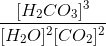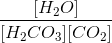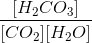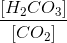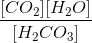# SAT II Chemistry : Reactions and Equilibrium

## Example Questions

### Example Question #1 : Identifying And Defining Acids And Bases

Which of the following cannot act as a Bronsted-Lowry base (proton recipient) in aqueous solution?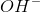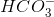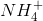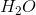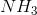Explanation:cannot receive another proton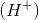because then it would become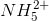, which does not exist. All of the other answer choices are fine: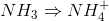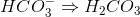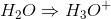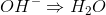### Example Question #1 : Reactions And Equilibrium

A scientist makes a solution by adding 0.2 grams of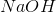to enough water so that the resulting solution has a volume of 10 liters. What, approximately, is the pH of this solution?Explanation:has a molar mass of approximately 40 g/mol, meaning that there is 0.01 mol of it in the solution. Sodium hydroxide is a strong base and completely dissociates in water. Its concentration in the solution is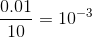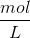. This means that the concentration of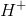ions is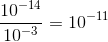and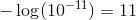. Thus, the pH of the solution is 11.

### Example Question #1 : Oxidation State

What is the oxidation number of nitrogen in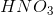?Explanation:

First, note that the molecule does not have a charge, meaning that the oxidation numbers of each atom must add up to zero. Hydrogen has an oxidation number ofand oxygen has an oxidation number of. Thus, if we call the oxidation number of nitrogen, we can get the equation. Solving this gives, so the oxidation number of nitrogen isin this molecule.

### Example Question #1 : Reaction Types

The following is a modified true/false question. In it, you must decide if each individual statement is true (T) or false (F). If both are true, then you must also decide if the second statement is a correct explanation (CE) of the first statement.

I: Anion would undergo reduction to formmetal

BECAUSE

II: reduction is a loss of electrons

T, F

T, T (Statement II is not a correct explanation of Statement I)

T, T, CE

F, T

F, F

T, F

Explanation:

Statement II is false: reduction is a gain of electrons. Looking at statement I, the ion's charge would decrease by 3 in becoming a metal, which suggests that the ion gains 3 electrons. Thus, it is undergoing reduction.

### Example Question #1 : Reactions And Equilibrium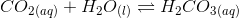What is an expression for the equilibrium constant for the above equation?